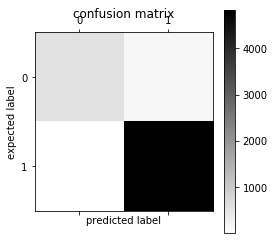# Spam Ham filter using sklearn¶

Naive Bayes classifiers are a popular statistical technique of e-mail filtering. They typically use bag of words features to identify spam e-mail, an approach commonly used in text classification.

Naive Bayes classifiers work by correlating the use of tokens (typically words, or sometimes other things), with spam and non-spam e-mails and then using Bayes' theorem to calculate a probability that an email is or is not spam.

Naive Bayes spam filtering is a baseline technique for dealing with spam that can tailor itself to the email needs of individual users and give low false positive spam detection rates that are generally acceptable to users. It is one of the oldest ways of doing spam filtering, with roots in the 1990s.

In :
import warnings
warnings.filterwarnings("ignore")

In :
%matplotlib inline
import matplotlib.pyplot as plt
import csv
import pandas as pd
import sklearn
import numpy as np
from sklearn.feature_extraction.text import CountVectorizer, TfidfTransformer
from sklearn.naive_bayes import MultinomialNB
from sklearn.svm import SVC, LinearSVC
from sklearn.metrics import classification_report, f1_score, accuracy_score, confusion_matrix
from sklearn.pipeline import Pipeline
from sklearn.grid_search import GridSearchCV
from sklearn.cross_validation import StratifiedKFold, cross_val_score, train_test_split
from textblob import TextBlob
from wordcloud import WordCloud


## Data prepration

In :
df = pd.read_csv('./data/SMSSpamCollection' , sep='\t' , names=['status', 'message'])

In :
df.head()

Out:
status message
0 ham Go until jurong point, crazy.. Available only ...
1 ham Ok lar... Joking wif u oni...
2 spam Free entry in 2 a wkly comp to win FA Cup fina...
3 ham U dun say so early hor... U c already then say...
4 ham Nah I don't think he goes to usf, he lives aro...
In :
len(df)

Out:
5572
In :
len(df[df.status=='spam'])

Out:
747

## Aggregate statistics analysis of data

Using pandas and matplotlib we can easily anlaysis the dataset

In :
df.groupby('status').describe()

Out:
message
count unique top freq
status
ham 4825 4516 Sorry, I'll call later 30
spam 747 653 Please call our customer service representativ... 4

#### how long the message is ?¶

getting length of each message=>

In :
df['length'] = df['message'].map(lambda msg:len(msg))

Out:
status message length
0 ham Go until jurong point, crazy.. Available only ... 111
1 ham Ok lar... Joking wif u oni... 29
2 spam Free entry in 2 a wkly comp to win FA Cup fina... 155
3 ham U dun say so early hor... U c already then say... 49
4 ham Nah I don't think he goes to usf, he lives aro... 61
In :
df['length'].plot(bins=40,kind='hist',color='orange')

Out:
<matplotlib.axes._subplots.AxesSubplot at 0x7fb636aea748>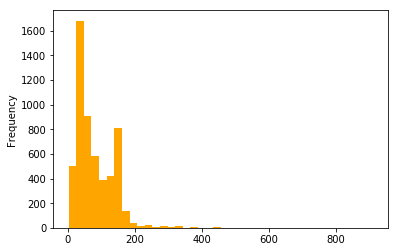In :
#describing message length
print(df['length'].describe())

count    5572.000000
mean       80.489950
std        59.942907
min         2.000000
25%        36.000000
50%        62.000000
75%       122.000000
max       910.000000
Name: length, dtype: float64

In :
#describing HAM message by length
print('HAM',df['length'].loc[df['status']=='ham'].describe())

HAM count    4825.000000
mean       71.482487
std        58.440652
min         2.000000
25%        33.000000
50%        52.000000
75%        93.000000
max       910.000000
Name: length, dtype: float64

In :
#describing SPAM message by length
print('SPAM',df['length'].loc[df['status']=='spam'].describe())

SPAM count    747.000000
mean     138.670683
std       28.873603
min       13.000000
25%      133.000000
50%      149.000000
75%      157.000000
max      223.000000
Name: length, dtype: float64

In :
df['length'].plot(kind='hist',bins=20,color='red',facecolor='green',alpha=0.5,normed=1)

Out:
<matplotlib.axes._subplots.AxesSubplot at 0x7fb63645bc88>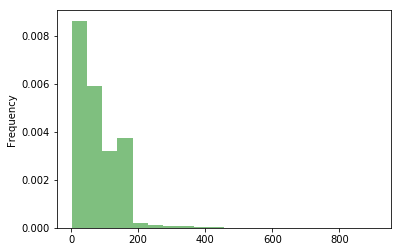In :
#length difference betwwn SPAM(1) and HAM(0)
df.hist(column='length', by='status', bins=20)

Out:
array([<matplotlib.axes._subplots.AxesSubplot object at 0x7fb6363dc0f0>,
<matplotlib.axes._subplots.AxesSubplot object at 0x7fb62ea15a58>], dtype=object)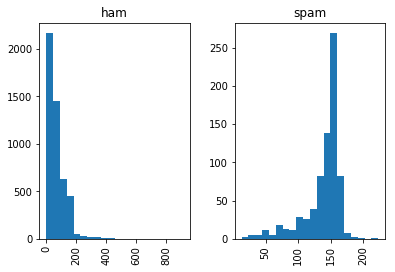#### getting largest and smallest message¶

In :
print("Largest messge is:",list(df.message[df.length>900]))  #we can get this 900 from above df.describe
print("Smallest message is ",list(df.message[df.length<3]))  #we can get this 3 from above df.describe

Largest messge is: ["For me the love should start with attraction.i should feel that I need her every time around me.she should be the first thing which comes in my thoughts.I would start the day and end it with her.she should be there every time I dream.love will be then when my every breath has her name.my life should happen around her.my life will be named to her.I would cry for her.will give all my happiness and take all her sorrows.I will be ready to fight with anyone for her.I will be in love when I will be doing the craziest things for her.love will be when I don't have to proove anyone that my girl is the most beautiful lady on the whole planet.I will always be singing praises for her.love will be when I start up making chicken curry and end up makiing sambar.life will be the most beautiful then.will get every morning and thank god for the day because she is with me.I would like to say a lot..will tell later.."]
Smallest message is  ['Ok', 'Ok', 'Ok', 'Ok']


## Data preprocessing

It is necessary because we can't apply any Machine learning model on text type data. We we need to tokenize the data. So we going to conver raw message into vectors.

#### Steps:¶

1. Split all data into invidual words
2. normalizing data
3. data to vectors

dependency:

first you need to downlaod punkt otherwise it will show you an error.
start python terminal and type
>>import nltk
>>nltk.download('punkt)
>>nltk.download('wordnet')

### Lemmatisation of data¶

The verb ' to go' may appear as 'go', 'goes', 'gone', 'going'. The base form, 'go', that one might look up in a dictionary, is called the lemma for the word.

In :
class Split:

"""This class is for spliting data into individual words and lemmatistion of words."""

def into_tokens(self,msg):
#         msg = unicode(msg, 'utf8')  # convert bytes into proper unicode
return TextBlob(msg).words

def into_lemmas(self,message):
#         message = unicode(message, 'utf8').lower()
words = TextBlob(message).words
# for each word, take its "base form" = lemma
return [word.lemma for word in words]

In :
split = Split()

Out:
0    [Go, until, jurong, point, crazy, Available, o...
1                       [Ok, lar, Joking, wif, u, oni]
2    [Free, entry, in, 2, a, wkly, comp, to, win, F...
3    [U, dun, say, so, early, hor, U, c, already, t...
4    [Nah, I, do, n't, think, he, goes, to, usf, he...
Name: message, dtype: object
In :
df.message.head().apply(split.into_lemmas)
# print(split_into_lemmas("go goes"))

Out:
0    [Go, until, jurong, point, crazy, Available, o...
1                       [Ok, lar, Joking, wif, u, oni]
2    [Free, entry, in, 2, a, wkly, comp, to, win, F...
3    [U, dun, say, so, early, hor, U, c, already, t...
4    [Nah, I, do, n't, think, he, go, to, usf, he, ...
Name: message, dtype: object

## What is text feature extraction?¶

Convert a collection of text documents to a matrix of token counts
Text Analysis is a major application field for machine learning algorithms. However the raw data, a sequence of symbols cannot be fed directly to the algorithms themselves as most of them expect numerical feature vectors with a fixed size rather than the raw text documents with variable length.

In :
vectorizer = CountVectorizer(analyzer=split.into_lemmas)

In :
#example
X = vectorizer.fit_transform(['hellow','hellow we are back','we we we are back'])
print(X)
# .vocabulary_ gives counts of all words in corpus
print(vectorizer.vocabulary_)

  (0, 2)	1
(1, 1)	1
(1, 0)	1
(1, 3)	1
(1, 2)	1
(2, 1)	1
(2, 0)	1
(2, 3)	3
{'hellow': 2, 'we': 3, 'are': 0, 'back': 1}

In :
X = vectorizer.fit_transform(df['message'])


getting feature names using vectorizer.get_feature_names

In :
vectorizer.get_feature_names()[:10]   #printing 10 feature

Out:
["''OK",
"'An",
"'Comfort",
"'HEART",
"'IF",
"'Luxury",
"'MARRIED",
"'Maangalyam",
"'Melle",
"'Need"]
In :
bag_of_words = vectorizer.fit_transform(df['message'])
X = vectorizer.transform(['win win win this'])
print(bag_of_words.shape,X.shape)

(5572, 11012) (1, 11012)


Now *bag_of_word* contains full SMS coupus in the from of vector

### Creating weigthage list using tf-idf using scikit-learn's TfidfTransformer¶

tf–idf or TFIDF, is short form of term frequency–inverse document frequency, is a numerical statistic that is intended to reflect how important a word is to a document in a collection or corpus. It is often used as a weighting factor in searches of information retrieval, text mining, and user modeling. The tf-idf value increases proportionally to the number of times a word appears in the document, but is often offset by the frequency of the word in the corpus, which helps to adjust for the fact that some words appear more frequently in general. Nowadays, tf-idf is one of the most popular term-weighting schemes.more

In :
tfidf_transformer = TfidfTransformer()
tfidf = tfidf_transformer.fit_transform(bag_of_words)
print(tfidf.shape)

(5572, 11012)

In :
df.loc[df["status"]=='ham',"status"]=1
df.loc[df["status"]=='spam',"status"]=0
df['status'] = df['status'].astype('int')


## Training model

Now we will train our classifier using numerical message vector.This is very easy using sklearn.

We will use MultinomialNB classifier of sklearn

In :
class Train:
"""Traning and testing our model."""

def __init__(self):
self.clf = MultinomialNB()

def train(self,x,y):
self.clf.fit(x,y)

def score(self,x,y):
return self.clf.score(x,y)

def test(self,y):
return self.clf.predict(y)

def accuracy(self,x,y):
acc = accuracy_score(x,y,normalize=False)
print('accuracy', acc)
return acc
def probability(self,x):
prob = self.clf.predict_proba(x)
return prob

In :
X_train, X_test, Y_train, Y_test = train_test_split(tfidf,df['status'],test_size=.1)
clf = Train()
clf.train(X_train,Y_train)

In :
print(clf.score(X_test,Y_test))

0.960573476703

In :
all_predictions = clf.test(tfidf)
a = clf.probability(tfidf)
print(a)
print(all_predictions)
print('accuracy', accuracy_score(df['status'], all_predictions))
print('confusion matrix\n', confusion_matrix(df['status'], all_predictions))
print('(row=expected, col=predicted)')

[[ -4.38487655e+00  -1.25427593e-02]
[ -4.61718328e+00  -9.92972467e-03]
[ -5.15432442e-02  -2.99099506e+00]
...,
[ -4.68641264e+00  -9.26246575e-03]
[ -7.56173919e+00  -5.20105361e-04]
[ -3.96738202e+00  -1.91042375e-02]]
[1 1 0 ..., 1 1 1]
accuracy 0.969490308686
confusion matrix
[[ 577  170]
[   0 4825]]
(row=expected, col=predicted)


### Testing on some random message string¶

In :
message = ['attending workshop']
X = vectorizer.transform(message)
print(X.shape)
clf.test(X)

(1, 11012)

Out:
array()

## Creating Word Cloud of Spam and Ham messages

In :
#spam  = 0 , Ham = 1 generating spam hand ham wordlist
spam_wl = ' '.join(list(df[df['status']==0]['message']))
ham_wl = ' '.join(list(df[df['status']==1]['message']))

In :
spam_wc = WordCloud(background_color='white',width=512,height=512).generate(spam_wl)
ham_wc = WordCloud(background_color='white',width=512,height=512).generate(ham_wl)


## Spam word cloud

In :
plt.figure(figsize=(10,8))
plt.imshow(spam_wc)

Out:
<matplotlib.image.AxesImage at 0x7fb62a005080>## Ham word cloud

In :
plt.figure(figsize=(10,8))
plt.imshow(ham_wc)

Out:
<matplotlib.image.AxesImage at 0x7fb62a15c128>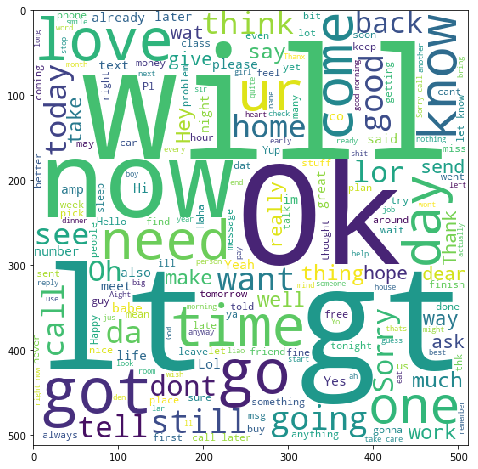### Getting classification report of Spam = 0 and Ham = 1¶

In :
print(classification_report(df['status'], all_predictions))

             precision    recall  f1-score   support

0       1.00      0.77      0.87       747
1       0.96      1.00      0.98      4825

avg / total       0.97      0.97      0.97      5572


In :
plt.matshow(confusion_matrix(df['status'], all_predictions), cmap=plt.cm.binary, interpolation='nearest')
plt.title('confusion matrix')
plt.colorbar()
plt.ylabel('expected label')
plt.xlabel('predicted label')

Out:
Text(0.5,0,'predicted label')